US: Tel: 1-206-279-3300
EU: Tel: +30 6986 007 252
• FREE VERSION OF RCsolver - Concrete Design with EC2, EC8, and ACI 318

Get full capabiltiies with our free version of RC-Solver once you signup for our newsletter. With the free version you will have to wait 30 seconds for advertisements.

# Correlation of the behavior factor member reinforcement details through the curvature ductility factor

The ductility classes medium and high (DCM and DCH) aim, through the seismic design, to control the post-elastic seismic behavior of the structure through the creation of a rigid and strong spine of vertical members, so that the inelastic deformations should be concentrated to the beam edges and to the base of the vertical members, and the formation of the areas of plastic joints so that they can develop plastic Tortional angles, compatible with the behavior factor q that is used in the design. In particular, these areas are formatted in order to have a curvature ductility factor equal to the displacement ductility factor of the building, μδ (Fardis, 2009a).

Moreover, ΕC8 (§5.2.3.4(3)) adopts the following equation between the curvature ductility factor, μφ on the edge of a member and the displacement ductility factor of this member, μδ:

μφ=2μδ-1

It can be easily proved that:

μφ=2q0-1                                        if  T1≥TC

μφ=1+2(q0-1) TC/T1 if  T1≤ TC

According to the previous value of the curvature ductility factor, μφ as it is chosen from the engineer and the two previous equations, we can calculate:

the maximum percentage of the tensile reinforcement on the flanges of the beams (EC8 §5.4.3.1.2(4)):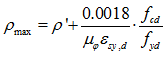where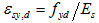is the design value of tension steel strain at yield and the reinforcement ratios of the tension and compression zone, ρ and ρ’. If the tension zone includes a slab, the amount of slab reinforcement parallel to the beam within the effective flange width is included in ρ.

• the mechanical volumetric ratio of confining hoops within the critical regions (i.e. : (a) to the base of columns and walls and (b) to the critical regions on the edges of columns in DCH that do not satisfy the capacity design of the member ). To the critical regions on the edges of columns in DCH that the capacity design of the member is satisfied, confining hoops are placed with mechanical volumetric ratio  that is calculated according to the reduced value of the curvature ductility factor, that is equivalent to a behavior factor value equal to 2/3 of the basic value qo.

The mechanical volumetric ratio of confining hoops  is given through the following equation: (EC8 §5.4.3.2.2(8))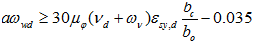where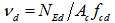is the normalized design axial force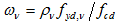the mechanical volumetric ratio of confining hoops within the web of the wall

bc is the gross cross-sectional width

bο is the width of the confined core (to the centerline of the hoops)

α    is the confinement effectiveness factor

# E-mail List Signup

Signup to our Email List for the latest information about our products, support and more.